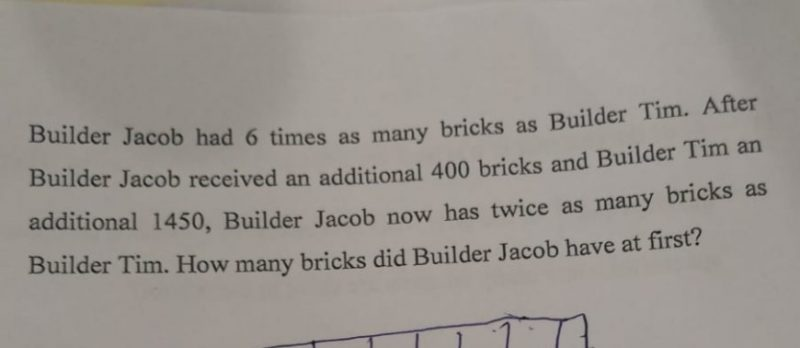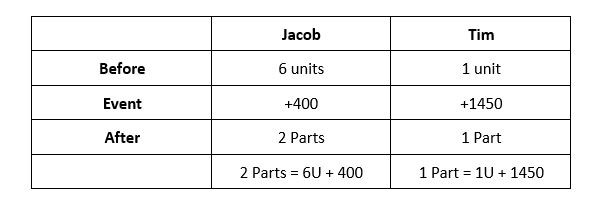QuestionHi,

P5 Math. Need help.

Thank you !2 Parts = 6U + 400

1 Part = 3U + 200

3U + 200 = 1 U + 1450

2U = 1450 – 200 = 1250

1U = 1250 ÷ 2 = 625

6U = 6 x 625 = 3750